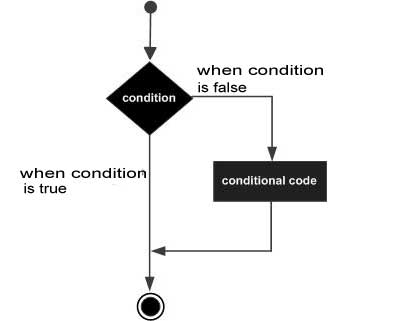# Perl unless Statement

A Perl unless statement consists of a boolean expression followed by one or more statements.

## Syntax

The syntax of an unless statement in Perl programming language is −

```unless(boolean_expression) {
# statement(s) will execute if the given condition is false
}
```

If the boolean expression evaluates to false, then the block of code inside the unless statement will be executed. If boolean expression evaluates to true then the first set of code after the end of the unless statement (after the closing curly brace) will be executed.

The number 0, the strings '0' and "" , the empty list () , and undef are all false in a boolean context and all other values are true. Negation of a true value by ! or not returns a special false value.

## Flow Diagram## Example

```#!/usr/local/bin/perl

\$a = 20;
# check the boolean condition using unless statement
unless( \$a < 20 ) {
# if condition is false then print the following
printf "a is not less than 20\n";
}
print "value of a is : \$a\n";

\$a = "";
# check the boolean condition using unless statement
unless ( \$a ) {
# if condition is false then print the following
printf "a has a false value\n";
}
print "value of a is : \$a\n";
```

First unless statement makes use of less than operator (<), which compares two operands and if first operand is less than the second one then it returns true otherwise it returns false. So when the above code is executed, it produces the following result −

```a is not less than 20
value of a is : 20
a has a false value
value of a is :
```
perl_conditions.htm